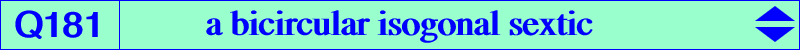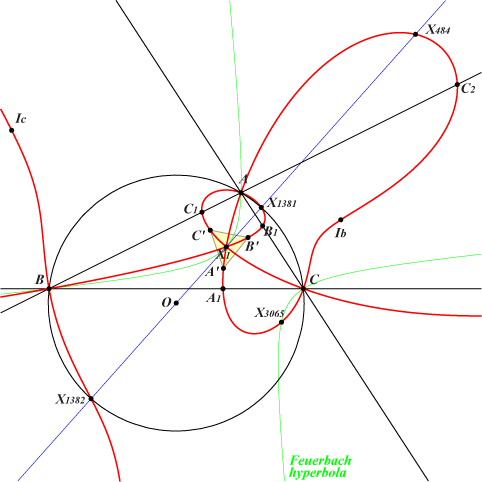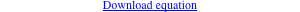too complicated to be written here. Click on the link to download a text file.X(1), X(484), X(1381), X(1382), X(3065), X(3307), X(3308), X(10215), X(10231) excenters A', B', C' : vertices of the BCI triangle (TCCT 6.39) other points belowThis (partly) answers a question from Dan Reznik : given a triangle ABC and a point P what is the locus of points X such that the reflection of the P-cevians about the X-cevians concurs ? (private message 2022-10-19). It is known that the reflections of the cevian lines of any point X in the corresponding internal bisectors of ABC (i.e. the cevian lines of I = X(1)) concur at the isogonal conjugate Y of X. On the other hand, the reflections of the cevians lines of X(1) in the cevian lines of X concur if and only if X lies on Q181. Q181 is a bicircular isogonal circum-sextic that passes through : • A, B, C which are nodes. The nodal tangents at A pass through the intersections of (O) and the line OIa, where Ia is the A-excenter of ABC. • X(1) which is a triple point with tangents parallel to the asymptotes of the McCay cubic K003. • the circular points at infinity J1, J2 which are nodes. The remaining points at infinity are X(3307), X(3308), on the Feuerbach hyperbola. • the incenters and excenters of triangles BCI, CAI, ABI. • A1, A2 on the sideline BC and on the circle passing through X(1), X(484) and A. The points B1, B2 on AC and C1, C2 on AB are defined cyclically. • A3, A4 on the external bisector at A and on the circle passing through X(1), X(484) whose center is on this same bisector and obviously on the perpendicular bisector of X(1), X(484). More generally, every circle passing through X(1), X(484), whose center is Ω, meets Q181 at 12 points, namely J1, J2 (each double), X(1) (triple), X(484) and two pairs of isogonal points that lie on two perpendicular lines secant at the isogonal conjugate gΩ of Ω, a point on the circum-conic with center X(10), perspector X(37). These two perpendicular lines are parallel to the asymptotes of the rectangular circum-hyperbola which is the isogonal transform of the perpendicular at O to the line ΩX(1). *** Generalization When the incenter X(1) is replaced by a fixed point P not lying on a sideline of ABC, the reflections of the cevians lines of P in the cevian lines of X concur if and only if X lies on a bicircular circum-sextic S(P) passing through the in/excenters. With P = X3, S(P) decomposes into the inversible circular quartic Q023 and the circumcircle. With P = X4 : S(P) decomposes into the antigonal circular quintic Q038 and the line at infinity. Each locus is the isogonal transform of the other. This holds for the two sextics S(P), S(P*) obtained when P, P* are two distinct isogonal conjugate points. At last, note that, when X is a given point, the locus of point P is generally a strophoid. See CL038. In particular, Q181 is the locus of nodes of non-isogonal circum-strophoids that pass through the incenter I = X(1).